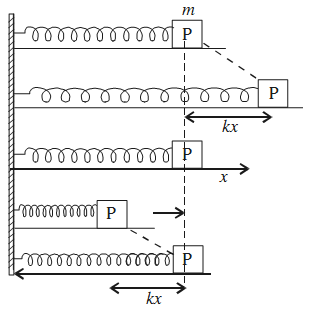# Oscillations of a Spring-Mass System

### Horizontal Oscillations

Consider a elastic spring of force constant k placed on a smooth horizontal surface and attached to a block P of mass m. The other end of the spring is attached to a rigid wall. Suppose that the mass of the spring is negligible in comparison to the mass of the block.Suppose that there is no loss of energy due to air resistance and friction.

Initially, at t = 0, the block is at rest and the spring is in relaxed condition. It is then pulled horizontally through a small distance. As the spring undergoes an extension x, it exerts a force on the block.

F = kx

The force is directed against the extension and tends to restore the block to its equilibrium position.

As the block returns to its initial position, it acquires a velocity v and hence a kinetic energy.

K = ½mv2
.
Owing to inertia of motion, the block overshoots the mean position and continues moving towards the left till it arrives at the certain position. In this position, the block again experiences a force kx which tries to bring it back to the initial position. In this way, the block continues oscillating about the mean position.

The time period of oscillation is

T = 2π√(m/k)

where k is the force per unit extension of the spring.

### Vertical Oscillations

Suspend a spring of force constant k from a rigid support. Then, attach a block of mass m to the free end of the spring. As a result of this, the spring undergoes an extension l.

The force constant of the spring is k = mg/l.

Pull down the block through a small distance, y. A force ky acts on the block vertically upwards. Therefore, on releasing the block, the force ky pulls it upwards. As the block returns to its initial position, it continues moving upwards on account of the velocity it has gained.

It overshoots the equilibrium position by a distance y. The compressed spring now applies on it a restoring force downwards. The block moves downwards and again overshoots the equilibrium position by almost the same vertical distance y. Thus, the system continues to execute vertical oscillations. The angular frequency of vertical oscillations is

ω = 2π/T = √(k/m)

T = 2π√(m/k)

Acceleration due to gravity does not influence vertical oscillations of a spring–mass system.